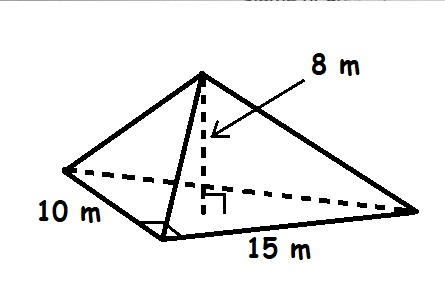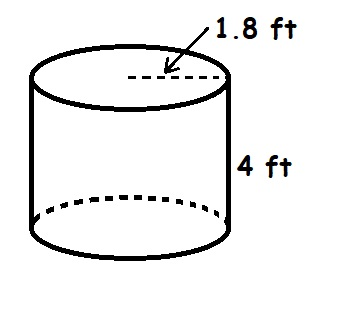# What is the volume of this triangular pyramid? FSP19610807261.jpgFSZ What is theeliaskidszs 2021-12-16 Answered
What is the volume of this triangular pyramid?What is the volume of this cylinder?
Use $\pi \approx 3.14$ and round your answer to the nearest hundredth.You can still ask an expert for help

• Questions are typically answered in as fast as 30 minutes

Solve your problem for the price of one coffee

• Math expert for every subject
• Pay only if we can solve itKirsten Davis

Step 1
In this question we will use basic of geometry i.e formula of volume of triangular pyramid and volume of cylinder.
We will use volume of triangular pyramid=$\frac{1}{3}$*Area of base *Height
Volume of cylinder $=\pi {R}^{2}h$
Solution of the above problem is in the step below.
(a)As we know the formula of Volume of triangular pyramid
So,
Volume of pyramid
As we can observe that
Area of base $=\frac{1}{2}×B×h=\frac{1}{2}×15×10=75{m}^{2}$
Height of triangular pyramid =8m
Hence,
Volume of pyramid $=\frac{1}{3}×75×8=200{m}^{3}$
Volume of triangular pyramid $=200{m}^{3}$

###### Not exactly what you’re looking for?puhnut1m
(b)As we know that the volume of cylinder $=\pi {r}^{2}h$
Where; r= radius of cylinder =1.8 ft
h= Height of cylinder =4 ft
So,
Volume of cylinder $=\pi {\left(1.8\right)}^{2}\left(4\right)$
Volume of cylinder $=40.6944f{t}^{3}$